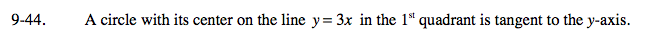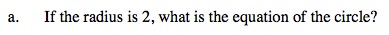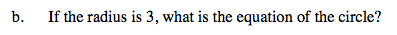### Home > A2C > Chapter 9 > Lesson 9.1.2 > Problem9-44

9-44.Try sketching the circle. The circle to the right has a radius of 2. Try placing it on the axis so it matches the circle described in the problem. The line y = 3x has been graphed for you.

Use the coordinates of the center and the radius to write an equation in general form.
(x − 2)2 + (y − 6)2 = 4See part (a).

(x − 3)2 + (y − 9)2 = 9

Use the eTool below to explore the problem further.
Click the link at right for the full version of the eTool: A2C 9-44 HW eTool